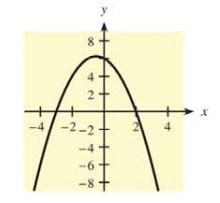Chapter 10.1, Problem 21E### Mathematical Applications for the ...

11th Edition
Ronald J. Harshbarger + 1 other
ISBN: 9781305108042

#### Solutions

Chapter
Section### Mathematical Applications for the ...

11th Edition
Ronald J. Harshbarger + 1 other
ISBN: 9781305108042
Textbook Problem

# For each function and graph in Problems 21-24(a) use the graph to identify x -values for which y ' >   0 , y ’ <   0 , y ' =   0 , and y’ does not exist.(b) use the derivative to check your conclusions. y = 6 − x − x 2(a)

To determine

The x-values where y>0,y<0 and y=0 for the function y=6xx2 using the graph and y does not exist.

Explanation

Given Information:

The provided function is y=6xx2.

The provided graph is:

Explanation:

Consider the provided graph:

The critical values are the only values at which the graph can have turning points, the derivative cannot change sign anywhere except at the critical value.

This, in an interval between two critical values, the sign of the derivative at any value in the interval will be the sign of the derivative at all values in the interval.

As per the First Derivative Test,

The first derivative of the function is evaluated. The first derivative is made equal to zero in order to get the critical points.

The values of the critical values are kept inside the original function which gives the critical points, which is x=12 in the above graph.

Thus, x=12 if y=0

Now, check the graph on left and right of x=12

On the left of x=12, graph is increasing,

(b)

To determine

To calculate: The derivative of the function y=6xx2 to check the conclusion of part (a).

### Still sussing out bartleby?

Check out a sample textbook solution.

See a sample solution

#### The Solution to Your Study Problems

Bartleby provides explanations to thousands of textbook problems written by our experts, many with advanced degrees!

Get Started

#### In Exercises 1124, find the indicated limits, if they exist. 24. limxx2x+1

Applied Calculus for the Managerial, Life, and Social Sciences: A Brief Approach

#### Calculate y'. 38. y=arctan(arcsinx)

Single Variable Calculus: Early Transcendentals, Volume I

#### Simplify: 1j6

Elementary Technical Mathematics